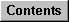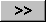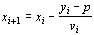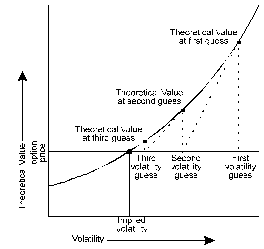Implied Volatility Formula

Implied volatility is a calculation that uses an options Vega (its sensitivity to change in volatility) to derive an estimate of volatility.

Formula:Where:

p = option price

xi = volatility

yi = options theoretical value at volatility xi

vi = options Vega at theoretical value yi

= degree of accuracy

The following figure illustrates the iterative process used to derive an options implied volatility.